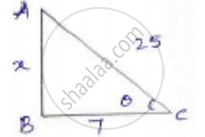Share

# In the Following, Trigonometric Ratios Are Given. Find the Values of the Other Trigonometric Ratios. Cos Theta = 7/25 - CBSE Class 10 - Mathematics

#### Question

In the following, trigonometric ratios are given. Find the values of the other trigonometric ratios.

cos theta = 7/25

#### Solution

We know that cos theta = "adjacent side"/"hypotence"

Now consider a right-angled Δle ABCLet x be the opposite side.

By applying Pythagoras theorem

𝐴𝐶2 = 𝐴𝐵2 + 𝐵𝐶2

(25)2 = 𝑥2 + 72

625 - 49 = 𝑥2

576 = sqrt576 = 24

sin theta = "opposite side"/"hypotenuse"= 24/25

tan theta = "opposite side"/"adjacent side" = 24/7

cosec theta = 1/sin theta = (1/3)/5 = 25/24

sec theta = 1/cos theta = (1/4)/5 = 25/7

cot theta = 1/tan theta = (1/3)/4 = 7/24

Is there an error in this question or solution?

#### APPEARS IN

Solution In the Following, Trigonometric Ratios Are Given. Find the Values of the Other Trigonometric Ratios. Cos Theta = 7/25 Concept: Trigonometric Ratios.
S